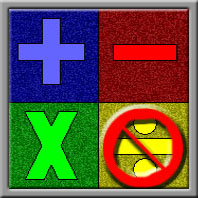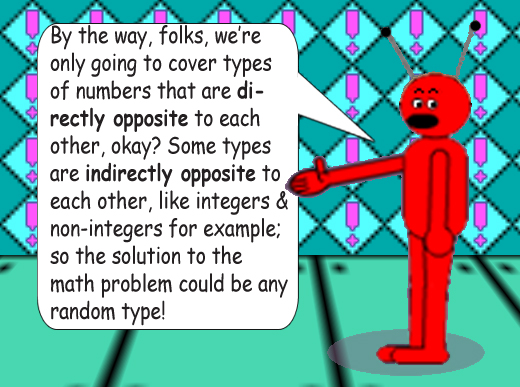# What Type of Number Will You Get?

This Web page is about the types of numbers you get as solutions/correct answers to math problems depending on the arithmetic operation used. (Except division; out of all 4 arithmetic operations, division is the most unpredictable & tends to gives you random types of quotients!) For more information about number types, see the page entitled "Types of Numbers".## Even × Odd = Even

(Multiplication is Commutative!)

## Positive × Negative = Negative

(Multiplication is Commutative!)

## Real × Imaginary = Imaginary

(Multiplication is Commutative!)

In conclusion, these are the only 3 different pairs of directly opposite number types because with indirectly opposite types, the number type of the solution is random! Multiplying an irrational number by an irrational number, for example, could give you either a rational product or an irrational product. The square root of 2 multiplied by itself gives you 2, which is rational; however, the square root of 2 multiplied by the square root of 3 = the square root of 6, which is irrational! Also, the square root of 2 + the square root of 3the square root of (2 + 3), which is 5; but yes, the sum of those 2 irrational numbers is also irrational; it's approximately equal to π + .0046717164... But adding 2 irrational numbers might give a rational number! (The square root of 2 + (1 - the square root of 2) = 1!)